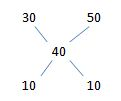# SSC CGL 2018 Practice Test Papers | Quantitative Aptitude (Day-11)

Dear Aspirants, Here we have given the Important SSC CGL Exam 2018 Practice Test Papers. Candidates those who are preparing for SSC CGL 2018 can practice these questions to get more confidence to Crack SSC CGL 2018 Examination.

[WpProQuiz 2803]

Click “Start Quiz” to attend these Questions and view Explanation

1). The average age of a woman and her twin sisters is 22 years. The ratio of ages of woman and her one sister is 13:10.Find the sum of the ages of twin sisters?

1. 30
2. 33
3. 25
4. 40

2). A distance 240 km is covered partially by bus and boat in 6 hours. The speed of bus is 30 kmph and the speed of boat is 50 kmph. Find the ratio of distances covered by bus to boat.

1. 1:1
2. 2:1
3. 3:5
4. 5:1

3). A certain sum of money is distributed among 15 people , each one gets Rs.51 and Rs. 35 is left. What is total amount?

1. 800
2. 350
3. 765
4. 1000

4). Two sofas J and K are sold at a profit of 20 % and 25 % respectively. If the profit is same, then what is the ratio of cost price of K to J?

1. 5:4
2. 4:5
3. 1:1
4. 2:3

5). A 60 litre solution contains water and Liquor in the ratio 2:3. How much liquor is to be taken out replaced with water to make the ratio 1:1?

1. 6 l
2. 12 l
3. 10  l
4. 5 l

6). 52001/60×29=?x41

1. 322
2. 456
3. 750
4. 650

7). If x+1/x=4 then x8+1/x8=?

a. 37634

b.30000

c.388

d.200

8). Sum of twice a fraction and its reciprocal is 57/20. What is the fraction?

a. 5/8

b. 4/5

c.3/2

d.4/6

9). If a3+b3=351 and ab=14. Find a+b?

a.9

b. 3

c. 10

d. 14

10). The compound ratio of 2/7, 5/6,1/5 and 7/9 is

a.1/13

b.1/27

c. 1/50

d.3/46

11). The hypotenuse of a right angled isosceles triangle is 18 cm Find the sum length of other two sides.

a.18√2

b.18

c.20

d.36

Sum of ages of 3 sisters=22×3=66

Ratio of their age=13:10:10

33x=66

X=2

Sum of ages of twin sisters=  20x=20*2=40

Average speed of bus and boat=(30+50)/2=40Ratio of time=1:1

Distance by bus =30×3=90

Distance by boat=50×3=150

Ratio of distance of bus to boat=90:150=3:5

Amount distributed=15×51=765

Total amount= 765+35=800

20% of CP of J= 25 % of CP of K

CP of (K/J)= 4/5

Ratio of water and liquor= 2:3

5x=60

X=12

Quantity of water=2x=2×12=24

Quantity of liquor=3x=3×12=36

(24+x)/(36-x)=1/1

X=6

2001/60×29=?x41

?=650

X+1/x=4

X2+1/X2=42-2=14

X4+1/X4=142-2=194

(X4+1/X4)(X4+1/X4)=x8+1/x8+2

x8+1/x8= 194×194-2=37634

Let the fraction be x And Its reciprocal be 1/x

2x+1/x=57/20

2x2+1=57x/20

40x2-57x+20=0

X=5/8

ab=14=>a=7,b=2

Substitute in a3+b3

73+23=343+8=351

Therefore, a+b=7+2=9

Compound ratio=2/7×5/6×1/5×7/9=1/27

In isosceles triangle two sides are equal.

Let x be the length of equal side

Hypotenuse of a right angled isosceles triangle=√2x=18

x=9√2

Sum=9√2+9√2=18√2